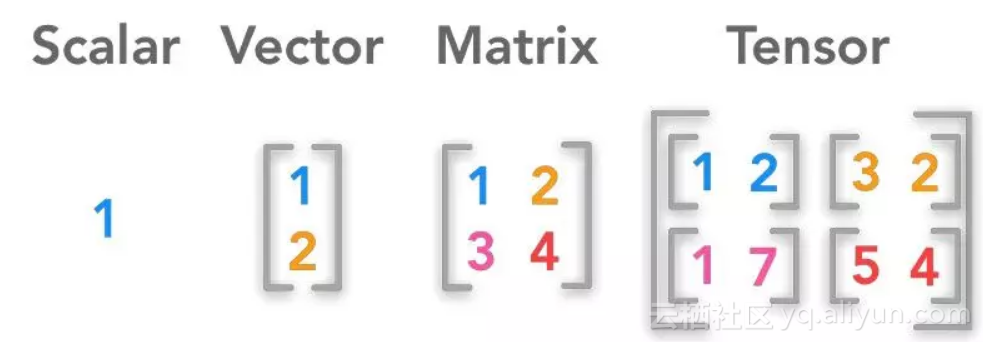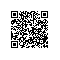# 一文解读Tensor到底是个啥玩意儿？（附代码）

（译者注：因为作者提到张量不仅包含数据，而且包含相应的操作，这与我们在面向对象编程中的约定是一致的，所以作者更建议理解成是对象。）import numpy as np

x = np.array(42)

print(x)

print('A scalar is of rank %d' %(x.ndim))

42

A scalar is of rank 0

x = np.array([1, 1, 2, 3, 5, 8])

print(x)

print('A vector is of rank %d' %(x.ndim))

[1 1 2 3 5 8]

A vector is of rank 1

## 矩阵

x = np.array([[1, 4, 7],

[2, 5, 8],

[3, 6, 9]])

print(x)

print('A matrix is of rank %d' %(x.ndim))

[[1 4 7]

[2 5 8]

[3 6 9]]

A matrix is of rank 2

x = np.array([[[1, 4, 7],

[2, 5, 8],

[3, 6, 9]],

[[10, 40, 70],

[20, 50, 80],

[30, 60, 90]],

[[100, 400, 700],

[200, 500, 800],

[300, 600, 900]]])

print(x)

print('This tensor is of rank %d' %(x.ndim))

[[[ 1 4 7]

[ 2 5 8]

[ 3 6 9]]

[[ 10 40 70]

[ 20 50 80]

[ 30 60 90]]

[[100 400 700]

[200 500 800]

[300 600 900]]]

This tensor is of rank 3